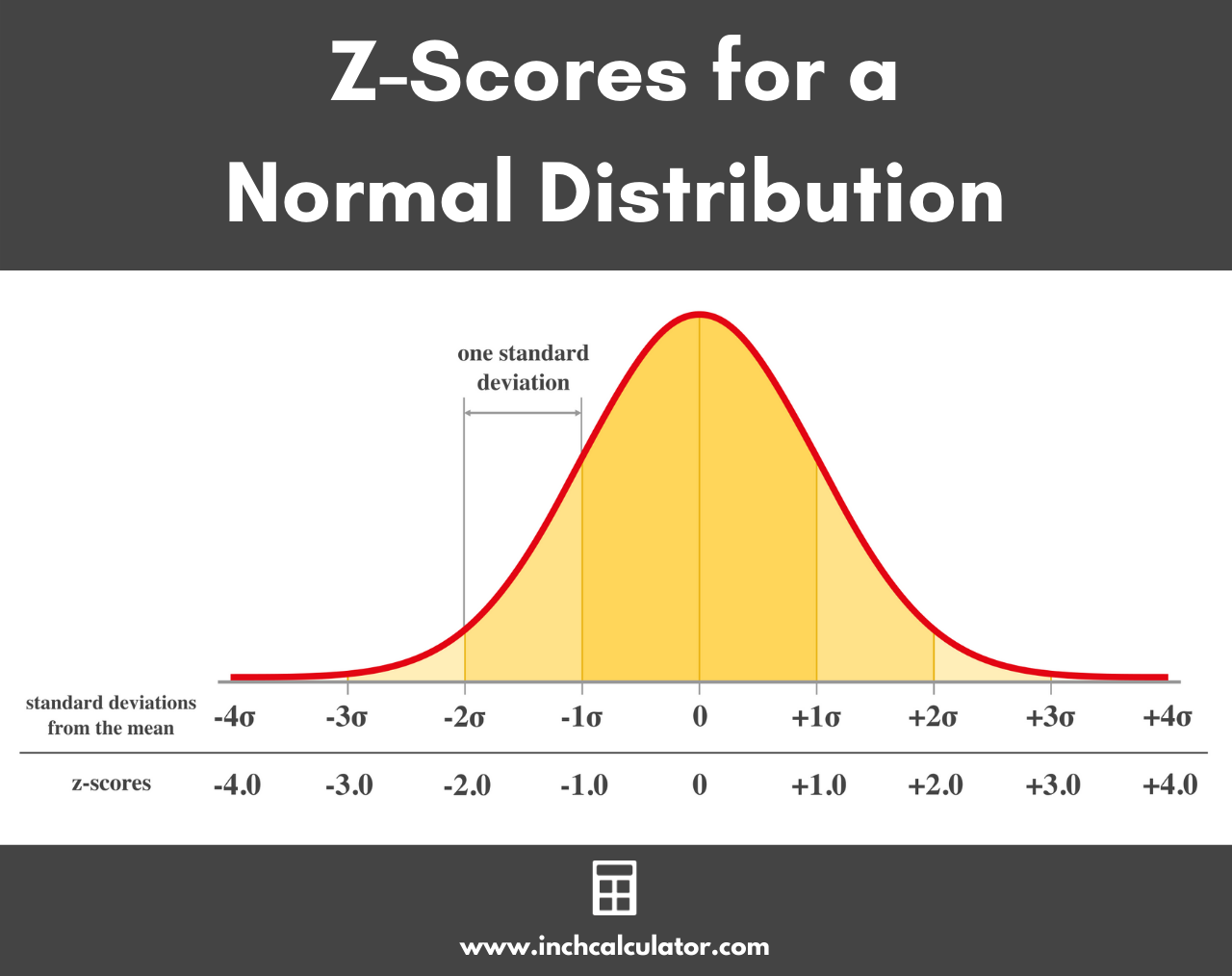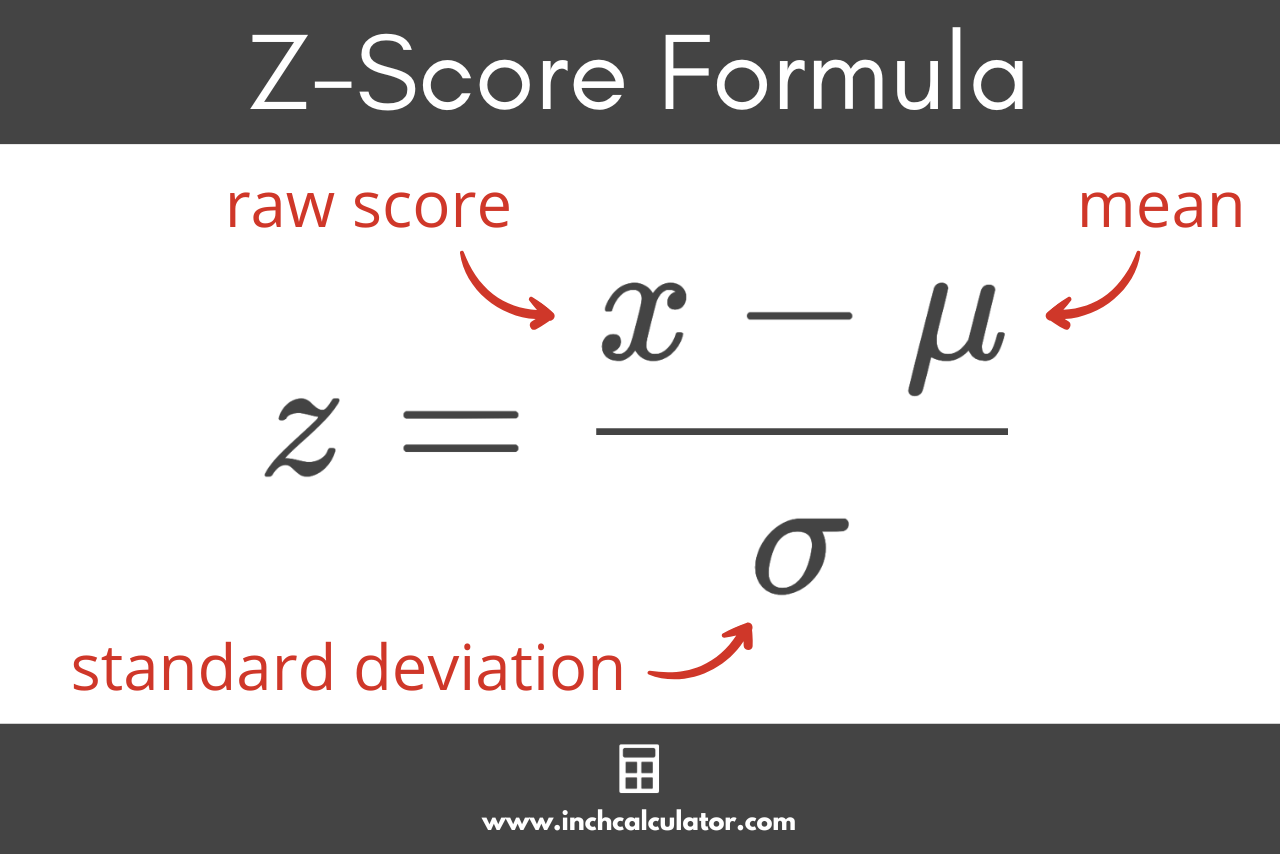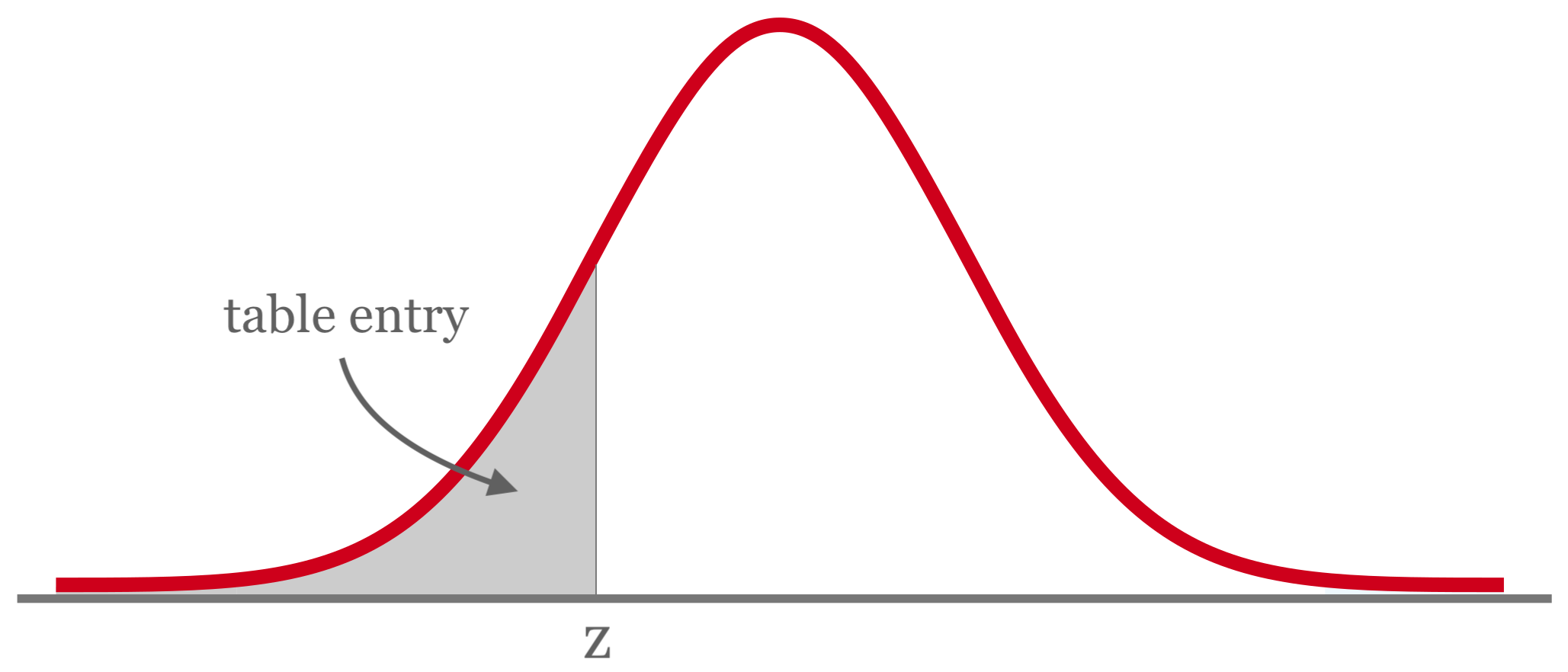# Z-Score Calculator

Enter the raw score, population mean, and standard deviation to find the z-score using the calculator below.

## Z-Score:

### Steps to Solve

#### Use the Z-Score Formula

z=\frac{x-\mu}{\sigma}

#### Substitute Values and Solve to Find the Z-Score

z=\ ?
Learn how we calculated this below

## How to Find the Z-Score

In statistics, the z-score, also called the standard score or normal score, is the number of standard deviations that a value is from the mean value. Each data point (aka raw score) in a normal distribution has a z-score, which is the number of standard deviations away from the mean of the population.Z-scores are useful statistics because they allow you to calculate the probability of a score in a standard normal distribution, also known as the p-value. You can also use it to calculate the margin of error in survey data, for example.

Of course, the easiest way to find the z-score is to use the calculator above, but you can also find it using a formula.

### Z-Score Formula

You can find the standard score for any value in a normal distribution using the z-score formula if you know the mean and standard deviation.

z=\frac{x-\mu}{\sigma}

Thus, the z-score z for a data point x is equal to x minus the population mean μ, divided by the standard deviation σ.### Steps to Find the Z-Score

You can find the z-score for a value in a few simple steps.

#### Step One: Find the Mean

The first step to finding the z-score is to find the mean for the population. You can use a mean calculator or use the mean formula.

\mu=\frac{\sum_{i=1}^{n}x_{i}}{n}

The population mean μ is equal to the sum of all of the values in the population xi, divided by the count of values in the population n.

#### Step Two: Find the Standard Deviation

The second step is to find the standard deviation for the data set. You can use a standard deviation calculator or use the standard deviation formula.

\sigma = \sqrt{\frac{\sum \left ( x_{i}-\mu \right )^{2}}{n}}

The standard deviation σ is equal to the square root of the sum of squares divided by the population size n, where the sum of squares is equal to the sum of each value minus the population mean squared.

#### Step Three: Find the Z-Score

The final step to finding the z-score is to use the formula above and substitute the values for the mean and standard deviation.

z=\frac{x-\mu}{\sigma}

For example, let’s say Jane was taking a statistics course, and she received a score of 93 on her final exam. The average score in the class was 89 and had a standard deviation of 3. Let’s calculate the z-score for Jane’s score.

z=\frac{\text{Jane’s score}-\text{average score}}{\text{standard deviation}}
z=\frac{93-89}{3}
z=\frac{4}{3}
z=1.\overline{33}

Thus, the z-score for Jane’s exam score is equal to 1.33.

## How to Find the Z-Score for Sample Means

It’s also possible to calculate the z-score for a random sample of raw scores. The resulting score will be the distance in standard errors between the sample mean and population mean.

### Z-Score of a Sample Formula

z=\frac{\bar{x}-\mu}{\sigma / \sqrt{n}}

The z-score for the sample is equal to the sample mean minus the population mean μ, divided by the standard error of the mean, which is equal to the population standard deviation σ divided by the square root of the number of observations n in the sample.

This is very similar to the one-sample t-test formula.

## How to Interpret a Z-Score

As noted above, the z-score is equal to the distance of a value from the mean in standard deviations, but what does that actually tell us? There are a few things we can take away from the z-score after we calculate it.

First, a positive z-value means that the raw score is greater than the mean, while a negative z-value means that the raw score falls below the mean. A z value of 0 means that the raw score is equal to the mean.

A very large z-score also tells us that the raw score is unusual, while a smaller z-score indicates that it might fall closer to the middle of the distribution. A z of greater than 3 or less than -3 generally indicates that the raw score is an outlier.

## What is a Z Table?

You might have also heard of a z table. A z table is a table that allows you to find the probability of a value being to the left of a z-score in a normal distribution.

Each entry in the z table represents the area under the normal distribution bell curve to the left of z. These are the left-tailed p values for the z-score.A z table is also sometimes called a standard normal table.